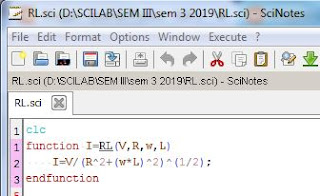LR Circuit using scilab

AIM OF THE EXPERIMENT:

To find the current through a LR circuit.

REQUISITES:

A PC/laptop.

Scilab 6.0.0 must be installed.

THEORY:

By knowing the emf, resistance & inductance of a LR circuit, we can find out the current flowing through it. The formula is given as

PROCEDURE:
2. Go to all programs & open scilab 6.0.0.
3. Go to Scinotes.
4. Write the coding/program.
5. Save the file & use extension name .sci
6. Then execute & go to the scilab console window for output.
7. Write I=LR(1,2,3,4) in the scilab console window.
8. Press enter to get the output.
CODING:
```clc

function I=RL(V, R, w, L)    ```
```I=V/(R^2+(w*L)^2)^(1/2);
endfunction```
```
```
``````
```
```
```
```
```
```
```
```
```
```
```
```
```
```
`OUTPUT:`
``````
```
```

```
Scilab Programming

C ++ Programming

Physics Notes: CLICK

IIT-JAM             JEST                GATE               NET

```